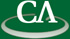### Computing the greatest common divisor of multivariate polynomials over finite fields.

#### Suling Yang, Mathematics, SFU.

Wednesday April 22nd, 3:30pm, in K9509.

```Abstract:

Richard Zippel's sparse modular GCD algorithm is widely used to
compute the monic greatest common divisor (GCD) of two multivariate
polynomials over the integers.  In this report, we present how this
algorithm can be modified to solve the GCD problem for polynomials
over finite fields of small cardinality. When the GCD is not monic,
Zippel's algorithm cannot be applied unless the normalization
problem is resolved. Alan Wittkopf et al. developed the LINZIP
algorithm for solving the normalization problem.
Mahdi Javadi proposed a refinement to the LINZIP algorithm.
We implemented his approach and will show that it is efficient
and effective on polynomials over small finite fields.
Zippel's algorithm also uses properties of transposed Vandermonde systems
to reduce the time and space complexity of his algorithm.  We also
investigated how this can be applied to our case.

```